可随意扭转的吧椅代替了剧场式的正襟危坐，庄重的信息发布变成了轻松的彼此沟通，如无特别介绍分不清谁为主谁是宾，若不看指示标牌你会感觉误入别人的大趴儿。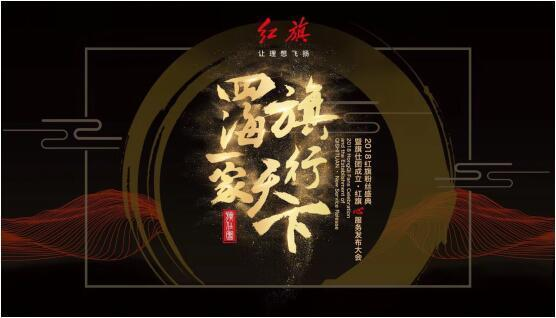印象中，这样的会议形式很难与红旗沾边儿，但这却实实在在就是“2018红旗粉丝盛典暨旗仕团成立·红旗心服务发布大会”的现场。
12月26日，冬至第四天，尽管长春已进入最寒冷的季节，但一场特别显然又是精心设计的聚会，却让远道而来的200多位红旗车主和粉丝如沐春风，也让人们看到了国车红旗的新气象。
“国车”诚意为“民”
于国人而言，红旗是独一无二的特殊存在。作为中国汽车产业的真正原创，红旗承担着与生俱来的责任和使命，红旗不仅是一款汽车产品，更是一种民族精神的象征，一种社会价值的体现。
进入新时代，红旗踏上了充分参与竞争的市场化之路。而红旗独特的中国格调与文化底蕴，也成为那些追求高尚精致生活、充满情怀、铁肩担义人士用来彰显民族骄傲与生活自信的最好载体。
与时代同行，与秉持相同价值观者同行，粉丝热爱红旗，也被红旗所热爱。粉丝不竭余力为红旗鼓与呼，红旗也在尽最大诚意为粉丝打造最尊贵的体验和享受——“旗仕团”及“心服务”应运而生。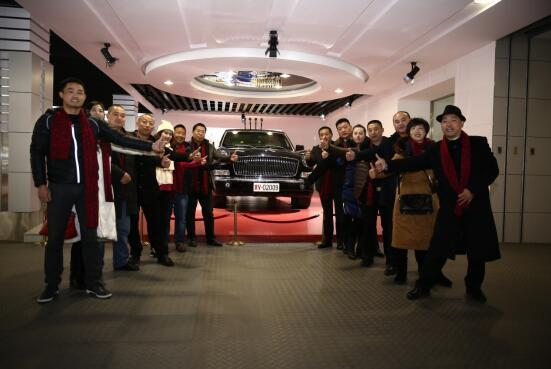作为传递红旗精神的阵地，红旗车主及粉丝共享精彩生活的家园，旗仕团被赋予了美好愿景：携手志同道合，激荡浩然正气，致敬高尚精致生活。并确定了将个人与他人无隙融合的行动纲领：文明驾驶，和谐出行，做中国好车主；家国情怀，敬业乐群，做社会好栋梁；心系至亲，天伦之乐，做家庭好成员；高尚精致，积极健康，做生活好旗仕。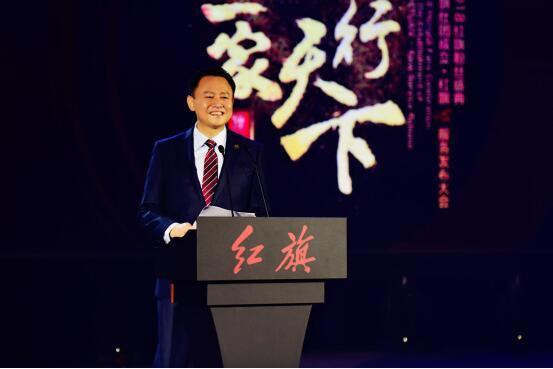中国第一汽车集团有限公司董事长徐留平对旗仕团寄予了殷殷期待：“作为心存家国情怀的品牌与人群，期望通过旗仕团，让红旗与粉丝一起打造一种高尚精致的生活格调，塑造一种积极健康的生活方式，传播一种自信向上的力量，建造一个传承中国优秀文化、传递新时代中国精神的家园”。
红旗“心服务”由“心安保障”、“心享体验”和“心动科技”三部分组成，贯穿了客户从看车、选车、用车乃至换车的全方位汽车服务链。其中，终身免费保修、终身免费救援、终身免费取送服务、+1二手车保值计划；100家红旗线下体验中心、数字体验中心、旗仕团、“一键智享服务”、红旗云店、智能网联车，以及包括维修质量、价格、效率三要素在内的“客户基础需求满足”，无一不是在以最大化满足客户需求为原点，让客户享受无与伦比的尊崇体验。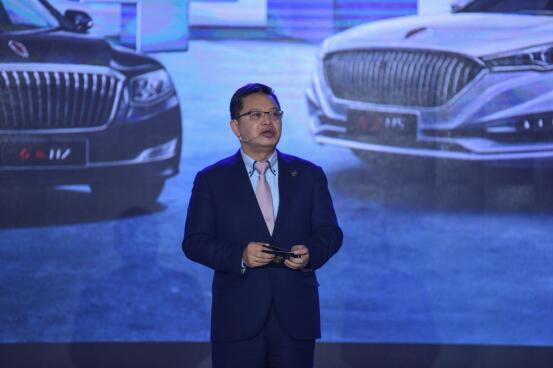中国第一汽车集团有限公司总经理助理、红旗销售公司总经理况锦文满怀深情地说：“‘心’字表达了我们对客户的‘真诚用心，感恩倾心’。红旗将全力打造一个‘用心’服务客户，有温度的服务品牌”。
营销只为与你更近
实际上，从面向民用市场销售的那一天起，红旗就对用户抱有足够热情，但由于本身时代烙印太过深刻，加之无经验可循的营销探索，使得红旗与普通人之间始终有一定距离，甚至是一道鸿沟。而消弭这道障碍的最有效途径，就是制定并有效贯彻可以被目标群体真心接受和认可、充分拉近与消费者距离的营销策略。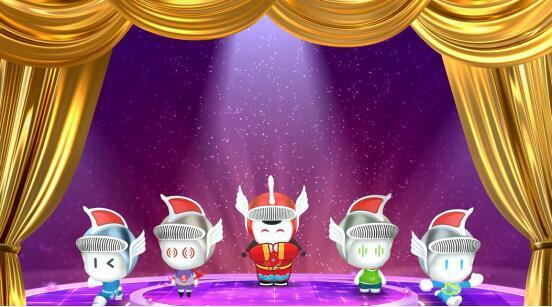如开篇所述，若将本次粉丝大会视为一次营销，那红旗这次展现出了大不同，也让人们看到了品牌新气象。除主要领导登台发布服务车主及粉丝的利好福利外，本次大会几乎是客户的舞台。尤其是旗仕团吉祥物“旗宝儿”的亮相，更引得众人会心而笑，让人瞬间觉得置身一场卡通人物见面会；即便是表达红旗文化自信与坚守中国格调决心的方式，都是依托一场近10分钟的模特T台秀。由此，一个可亲又不失格调、时尚又不乏底蕴的红旗形象被亲临现场的每一位参与者所感知，所认同。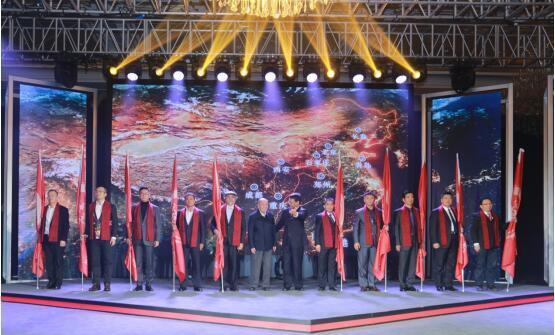论规格而言，本次大会虽未邀请媒体到场，但中国第一汽车集团有限公司董事长徐留平，中国一汽与红旗品牌相关的主要领导，以及红旗品牌营销团队主要负责人，甚至是关心红旗成长的退休老领导均悉数出席，人员涉及面之广佐证了会议规格之高。这让与会红旗车主与粉丝都大感意外，也体现出以人为本、以客为尊的红旗态度。
态度背后也折射出红旗营销的睿智考量。显然，分享与共建是网络时代品牌建设最重要的关键词，而粉丝盛典则可让红旗通过“粉丝效应”与消费者建立一种更有粘性的互动关系，这将以利于更多人接触、认识红旗，进而熟知、传播红旗。同时，旗仕团作为一个粉丝社群组织，更是一个红旗与粉丝可以形成分享式的、“多对多”的沟通载体，且其功能空间巨大，红旗在这里可以用更多有张力、有温度、有内涵、有细节的内容打动粉丝，与之形成共振、共鸣，进而激发病毒式的粉丝自传播。
中国第一汽车集团有限公司董事长徐留平宣布，2018年1月8日，红旗将在北京发布全新品牌战略，届时将系统阐释红旗的品牌理念、家族特征和产品谱系。本次粉丝盛典显然为之做了最好的铺垫和预热。可以说，红旗通过一场漂亮的外围战，一套成体系的组合拳，揭开了以人为本，以客为尊，遵循市场规律，有思路、有步骤的全新发展之路，也让人们看到了红旗打造中国第一且唯一豪华汽车品牌的新希望。

##### 相关资讯

|分享

•已阅！握爪
•我手滑为你点赞
•128个赞！
•不明觉厉
•阅后既醉
•有钱！任性
•照片太美，我不敢看
•干货！杠杠的
•高大上
•膜拜中！
•请接受我的膝盖
•猫哥/猫妹么么哒
•神吐槽
•我只笑笑不说话
•我想静静
•窒息
##### 资讯相关车系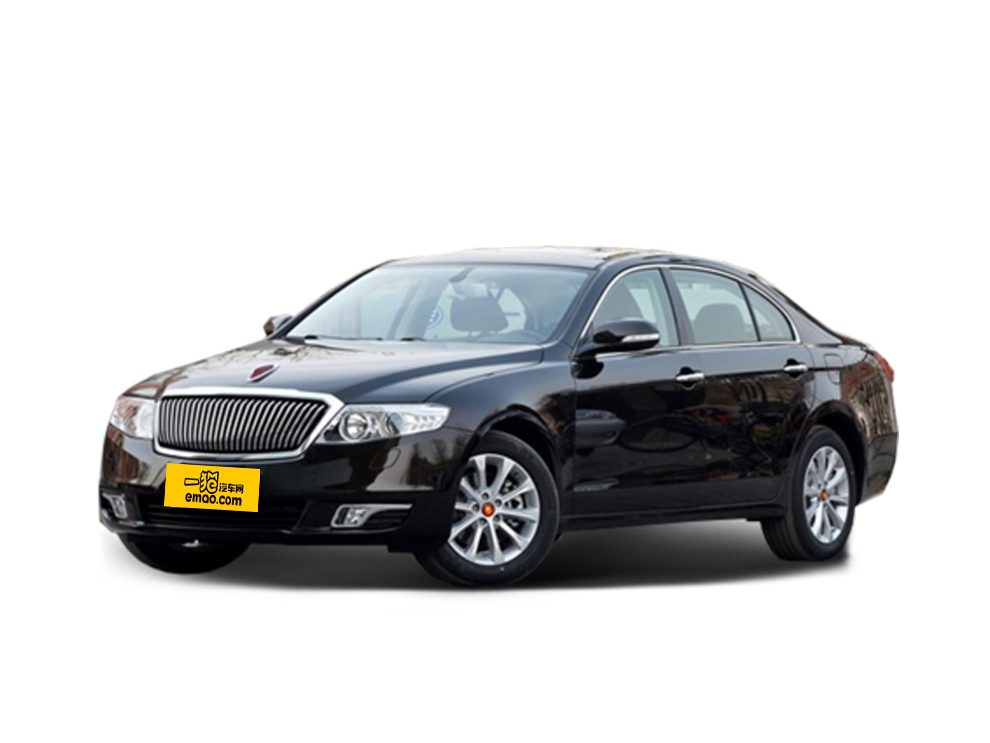##### 热度排行• 阿斯顿·马丁
• 奥迪
• 阿尔法罗密欧
• ALPINA

• 巴博斯
• 宝骏
• 宝马
• 保时捷
• 北汽制造
• 奔驰
• 奔腾
• 本田
• 比亚迪
• 标致
• 别克
• 宾利
• 布加迪
• 北汽威旺
• 北京
• 北汽绅宝
• 北汽幻速
• 北汽新能源
• 宝沃
• 比速汽车
• 北汽道达

• 昌河
• 长安
• 长城
• 长安商用
• 成功汽车
• 长江EV
• 长安轻车型

• 大众
• 道奇
• 东风
• 东风风神
• 东风小康
• 东南
• DS
• 东风风行
• 东风风度
• 东风风光

• 法拉利
• 菲亚特
• 丰田
• 福迪
• 福特
• 福田汽车
• 福汽启腾
• 风诺

• GMC
• 广汽传祺
• 广汽吉奥
• 观致

• 哈飞
• 海格
• 海马
• 华泰
• 黄海
• 恒天
• 红旗
• 哈弗
• 华颂
• 华凯
• 华泰新能源
• 汉腾汽车

• Jeep
• 江淮
• 江铃
• 捷豹
• 金杯
• 九龙
• 吉利汽车
• 金旅
• 金龙
• 江铃集团轻汽
• 江铃集团新能源
• 君马汽车
• 捷途

• 开瑞
• 凯迪拉克
• 科尼赛克
• 克莱斯勒
• KTM
• 卡威
• 凯翼
• 康迪
• 康迪电动汽车集团

• 猎豹汽车
• 兰博基尼
• 劳斯莱斯
• 雷克萨斯
• 雷诺
• 理念
• 力帆
• 莲花汽车
• 林肯
• 铃木
• 陆风
• 路虎
• 路特斯
• 领志
• 领克
• 零跑汽车

• MG
• MINI
• 马自达
• 玛莎拉蒂
• 迈凯伦
• 摩根

• 纳智捷

• 讴歌
• 欧宝
• 欧朗
• 欧拉
• 欧尚汽车

• 帕加尼

• 奇瑞
• 启辰
• 起亚
• 前途
• 庆铃汽车

• 日产
• 荣威
• 瑞麒汽车
• 如虎
• 瑞驰

• 上汽大通
• smart
• 三菱
• 双环
• 双龙
• 斯巴鲁
• 斯柯达
• 萨博
• 思铭
• 赛麟
• SWM斯威汽车

• TESLA
• 泰卡特
• 腾势

• 威麟
• 威兹曼
• 沃尔沃
• 五菱汽车
• 五十铃
• 潍柴英致
• WEY
• 蔚来
• 威马汽车

• 现代
• 雪佛兰
• 雪铁龙
• 西雅特
• 新特汽车
• 小鹏汽车
• 新宝骏

• 野马汽车
• 一汽
• 依维柯
• 英菲尼迪
• 永源
• 驭胜

• 中华
• 中兴
• 众泰
• 知豆
• 之诺
• 正道汽车
• A
• B
• C
• D
• E
• F
• G
• H
• I
• J
• K
• L
• M
• N
• O
• P
• Q
• R
• S
• T
• U
• V
• W
• X
• Y
• Z

• 阿斯顿·马丁
• 奥迪
• 阿尔法罗密欧
• ALPINA

• 巴博斯
• 宝骏
• 宝马
• 保时捷
• 北汽制造
• 奔驰
• 奔腾
• 本田
• 比亚迪
• 标致
• 别克
• 宾利
• 布加迪
• 北汽威旺
• 北京
• 北汽绅宝
• 北汽幻速
• 北汽新能源
• 宝沃
• 比速汽车
• 北汽道达

• 昌河
• 长安
• 长城
• 长安商用
• 成功汽车
• 长江EV
• 长安轻车型

• 大众
• 道奇
• 东风
• 东风风神
• 东风小康
• 东南
• DS
• 东风风行
• 东风风度
• 东风风光

• 法拉利
• 菲亚特
• 丰田
• 福迪
• 福特
• 福田汽车
• 福汽启腾
• 风诺

• GMC
• 广汽传祺
• 广汽吉奥
• 观致

• 哈飞
• 海格
• 海马
• 华泰
• 黄海
• 恒天
• 红旗
• 哈弗
• 华颂
• 华凯
• 华泰新能源
• 汉腾汽车

• Jeep
• 江淮
• 江铃
• 捷豹
• 金杯
• 九龙
• 吉利汽车
• 金旅
• 金龙
• 江铃集团轻汽
• 江铃集团新能源
• 君马汽车
• 捷途

• 开瑞
• 凯迪拉克
• 科尼赛克
• 克莱斯勒
• KTM
• 卡威
• 凯翼
• 康迪
• 康迪电动汽车集团

• 猎豹汽车
• 兰博基尼
• 劳斯莱斯
• 雷克萨斯
• 雷诺
• 理念
• 力帆
• 莲花汽车
• 林肯
• 铃木
• 陆风
• 路虎
• 路特斯
• 领志
• 领克
• 零跑汽车

• MG
• MINI
• 马自达
• 玛莎拉蒂
• 迈凯伦
• 摩根

• 纳智捷

• 讴歌
• 欧宝
• 欧朗
• 欧拉
• 欧尚汽车

• 帕加尼

• 奇瑞
• 启辰
• 起亚
• 前途
• 庆铃汽车

• 日产
• 荣威
• 瑞麒汽车
• 如虎
• 瑞驰

• 上汽大通
• smart
• 三菱
• 双环
• 双龙
• 斯巴鲁
• 斯柯达
• 萨博
• 思铭
• 赛麟
• SWM斯威汽车

• TESLA
• 泰卡特
• 腾势

• 威麟
• 威兹曼
• 沃尔沃
• 五菱汽车
• 五十铃
• 潍柴英致
• WEY
• 蔚来
• 威马汽车

• 现代
• 雪佛兰
• 雪铁龙
• 西雅特
• 新特汽车
• 小鹏汽车
• 新宝骏

• 野马汽车
• 一汽
• 依维柯
• 英菲尼迪
• 永源
• 驭胜

• 中华
• 中兴
• 众泰
• 知豆
• 之诺
• 正道汽车
• A
• B
• C
• D
• E
• F
• G
• H
• I
• J
• K
• L
• M
• N
• O
• P
• Q
• R
• S
• T
• U
• V
• W
• X
• Y
• Z

• 阿斯顿·马丁
• 奥迪
• 阿尔法罗密欧
• ALPINA

• 巴博斯
• 宝骏
• 宝马
• 保时捷
• 北汽制造
• 奔驰
• 奔腾
• 本田
• 比亚迪
• 标致
• 别克
• 宾利
• 布加迪
• 北汽威旺
• 北京
• 北汽绅宝
• 北汽幻速
• 北汽新能源
• 宝沃
• 比速汽车
• 北汽道达

• 昌河
• 长安
• 长城
• 长安商用
• 成功汽车
• 长江EV
• 长安轻车型

• 大众
• 道奇
• 东风
• 东风风神
• 东风小康
• 东南
• DS
• 东风风行
• 东风风度
• 东风风光

• 法拉利
• 菲亚特
• 丰田
• 福迪
• 福特
• 福田汽车
• 福汽启腾
• 风诺

• GMC
• 广汽传祺
• 广汽吉奥
• 观致

• 哈飞
• 海格
• 海马
• 华泰
• 黄海
• 恒天
• 红旗
• 哈弗
• 华颂
• 华凯
• 华泰新能源
• 汉腾汽车

• Jeep
• 江淮
• 江铃
• 捷豹
• 金杯
• 九龙
• 吉利汽车
• 金旅
• 金龙
• 江铃集团轻汽
• 江铃集团新能源
• 君马汽车
• 捷途

• 开瑞
• 凯迪拉克
• 科尼赛克
• 克莱斯勒
• KTM
• 卡威
• 凯翼
• 康迪
• 康迪电动汽车集团

• 猎豹汽车
• 兰博基尼
• 劳斯莱斯
• 雷克萨斯
• 雷诺
• 理念
• 力帆
• 莲花汽车
• 林肯
• 铃木
• 陆风
• 路虎
• 路特斯
• 领志
• 领克
• 零跑汽车

• MG
• MINI
• 马自达
• 玛莎拉蒂
• 迈凯伦
• 摩根

• 纳智捷

• 讴歌
• 欧宝
• 欧朗
• 欧拉
• 欧尚汽车

• 帕加尼

• 奇瑞
• 启辰
• 起亚
• 前途
• 庆铃汽车

• 日产
• 荣威
• 瑞麒汽车
• 如虎
• 瑞驰

• 上汽大通
• smart
• 三菱
• 双环
• 双龙
• 斯巴鲁
• 斯柯达
• 萨博
• 思铭
• 赛麟
• SWM斯威汽车

• TESLA
• 泰卡特
• 腾势

• 威麟
• 威兹曼
• 沃尔沃
• 五菱汽车
• 五十铃
• 潍柴英致
• WEY
• 蔚来
• 威马汽车

• 现代
• 雪佛兰
• 雪铁龙
• 西雅特
• 新特汽车
• 小鹏汽车
• 新宝骏

• 野马汽车
• 一汽
• 依维柯
• 英菲尼迪
• 永源
• 驭胜

• 中华
• 中兴
• 众泰
• 知豆
• 之诺
• 正道汽车
• A
• B
• C
• D
• E
• F
• G
• H
• I
• J
• K
• L
• M
• N
• O
• P
• Q
• R
• S
• T
• U
• V
• W
• X
• Y
• Z